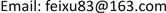1. 引言

2. 实验原理

θ ≈ tan θ = Δ l K (1)

tan 2 θ ≈ 2 θ = b D (2)

Δ l = K b 2 D (3)

Y = F l S Δ l (4)

3. 实验存在的误差分析3.1. 仪器误差分析

3.2. 实验操作的误差

4. 设计方案4.1. 实验装置的改进方案

θ ≈ tan θ = Δ l K (5)

tan 6 θ ≈ 6 θ = b D ′ (6)

Δ l = K ′ b 12 D ′ (7)

Y = F l S Δ l = 12 F l D ′ π d 2 K ′ b (8)

4.2. 实验操作方法的改进

4.3. 实验操作方法的改进 Measurement data of wire diamete

Measurement data of scale reading before device improvemen

Measurement data of scale reading after improvement of the devic

5. 结论